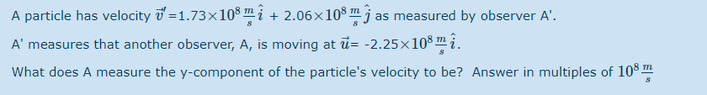JoeyBob
Homework Statement:
see attached
Relevant Equations:
u'=u/(δ(1-(uv)/c^2)
So for the formula, u'=u/(δ(1-(uv)/c^2)

u=2.06E8 and v=0. I am only looking at the y components here.

Since v=0 it really becomes u'=u/δ or u'= u*sqrt(1-(u^2)/c^2)).

Anyways when I plug this in I am getting 1.49E8 when the answer should be 0.951E8. Am I not using the correct formula here?

#### Attachments

•question.PNG
5.3 KB · Views: 49

Staff Emeritus
Homework Helper
There are three velocities in this problem. Are you sure you're using the right one when calculating ##\gamma##?

JoeyBob
There are three velocities in this problem. Are you sure you're using the right one when calculating ##\gamma##?
Its asking for the y component, which is j hat. The particle has a y-component velocity while the moving observer does not.

guv
Make sure you are taking care of the x direction relative velocity first; then you can work on the y direction relative velocity. You will need to perform relative velocity calculation twice. Keep in mind, relative motion has an effect on both time (indenpdent of direction) and space (dependent of direction).

JoeyBob
Make sure you are taking care of the x direction relative velocity first; then you can work on the y direction relative velocity. You will need to perform relative velocity calculation twice. Keep in mind, relative motion has an effect on both time (indenpdent of direction) and space (dependent of direction).
Why would I need to do x component first? Couldnt i just ignore x component and do y component since that's what the question is asking?

Staff Emeritus
Homework Helper
You're trying to find ##\vec v##, the velocity of the particle in the the rest frame of A. So why are you using the formula to find ##v_y'##? Also, you need to understand what the variables in the formula stand for. From your original attempt, it seems you have a misunderstanding there.

•PeroK
JoeyBob
You're trying to find ##\vec v##, the velocity of the particle in the the rest frame of A. So why are you using the formula to find ##v_y'##? Also, you need to understand what the variables in the formula stand for. From your original attempt, it seems you have a misunderstanding there.

u'=u/(δ(1-(uv)/c^2)

u' is the velocity of the object according to the individual moving at velocity v.

u is the velocity of the object according the the individual in the rest frame.

The question is asking for u`', the velocity of the object according to the individual moving at velocity v.

Am I incorrect anywhere in the above?

Except both individuals are at rest if we only look at the y-component.

Staff Emeritus
Homework Helper
It's a little confusing because you're mixing notation and reusing variables. According to A', the particle moves with velocity ##\vec v'##, so the usual convention is to say the particle moves with velocity ##\vec v## according to A.

Except both individuals are at rest if we only look at the y-component.
That's not how it works.

JoeyBob
That's not how it works.
How does it work then? I've determined the x-component of the velocity to be 2.7784E8 using ux'=(ux-v)/(1-(ux*v)/c^2) where I defined the variables in the same manner. i believe my answer is correct, though I have no way of veryfying.

An earlier comment said I needed to find the x-component first in order to find the y-component. The vital part I am missing is why that is the case.

Staff Emeritus
Homework Helper
You said
$$u_y'= \frac{u_y}{\gamma[1-(u_y v)/c^2]}.$$ If you write ##\gamma## in terms of ##v##, you have
$$u_y'= \frac{u_y\sqrt{1-(v/c)^2}}{1-(u_y v)/c^2}.$$ You set ##v=0## in the denominator but not in the numerator. Hopefully, it's obvious why you can't do that.

JoeyBob
You said
$$u_y'= \frac{u_y}{\gamma[1-(u_y v)/c^2]}.$$ If you write ##\gamma## in terms of ##v##, you have
$$u_y'= \frac{u_y\sqrt{1-(v/c)^2}}{1-(u_y v)/c^2}.$$ You set ##v=0## in the denominator but not in the numerator. Hopefully, it's obvious why you can't do that.
But if I do both numerator/denominator u'=u, which isn't the right answer.